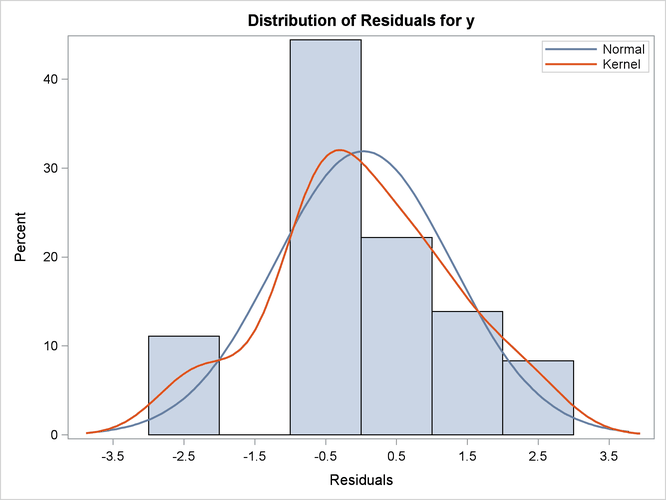The AUTOREG Procedure

Example 8.8 Illustration of ODS Graphics

This example illustrates the use of ODS GRAPHICS. This is a continuation of the section Forecasting Autoregressive Error Models.

These graphical displays are requested by specifying the ODS GRAPHICS statement. For information about the graphs available in the AUTOREG procedure, see the section ODS Graphics.

The following statements show how to generate ODS GRAPHICS plots with the AUTOREG procedure. In this case, all plots are requested using the ALL option in the PROC AUTOREG statement, in addition to the ODS GRAPHICS statement. The plots are displayed in Output 8.8.1 through Output 8.8.8. Note: these plots can be viewed in the Autoreg.Model.FitDiagnosticPlots category by selecting ViewResults.

```data a;
ul = 0; ull = 0;
do time = -10 to 36;
u = + 1.3 * ul - .5 * ull + 2*rannor(12346);
y = 10 + .5 * time + u;
if time > 0 then output;
ull = ul; ul = u;
end;
run;

data b;
y = .;
do time = 37 to 46; output; end;
run;

data b;
merge a b;
by time;
run;
```
```proc autoreg data=b all plots(unpack);
model y = time / nlag=2 method=ml;
output out=p p=yhat  pm=ytrend
lcl=lcl ucl=ucl;
run;
```

Output 8.8.1: Residuals Plot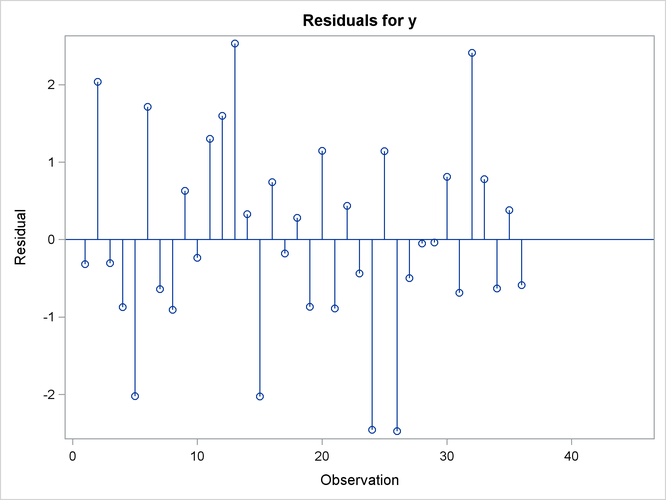Output 8.8.2: Predicted versus Actual Plot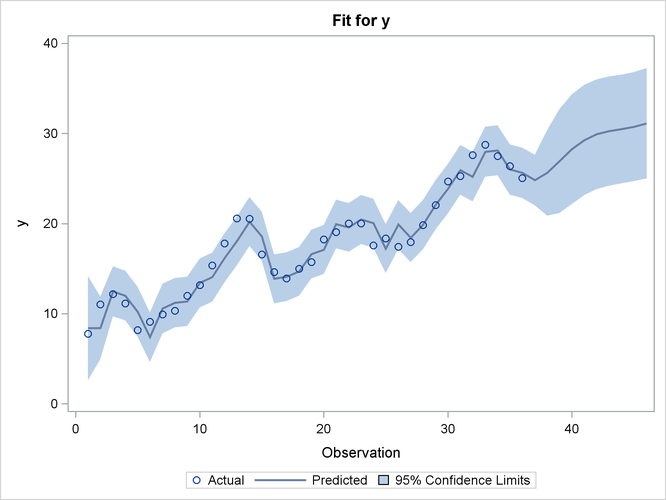Output 8.8.3: Autocorrelation of Residuals Plot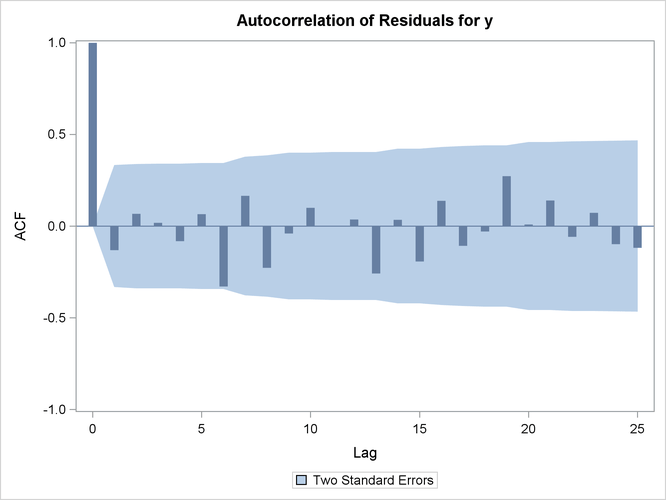Output 8.8.4: Partial Autocorrelation of Residuals Plot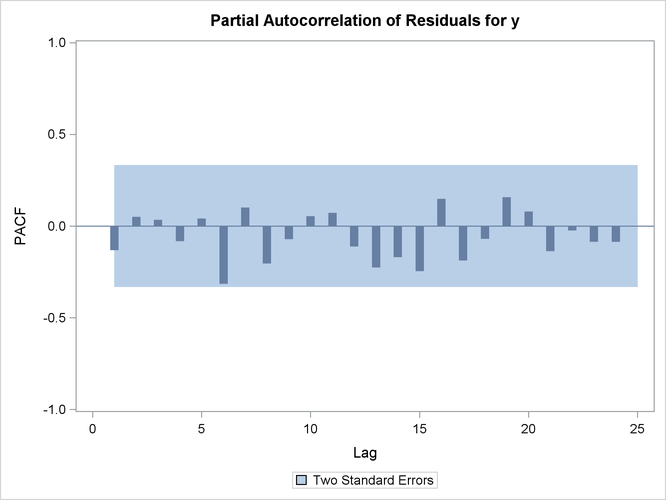Output 8.8.5: Inverse Autocorrelation of Residuals Plot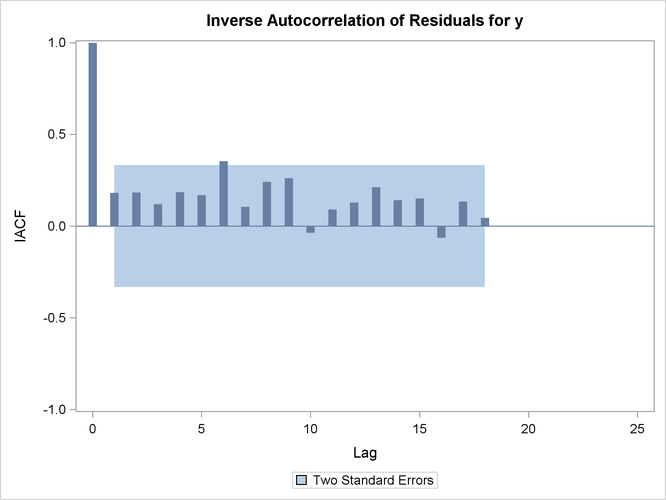Output 8.8.6: Tests for White Noise Residuals Plot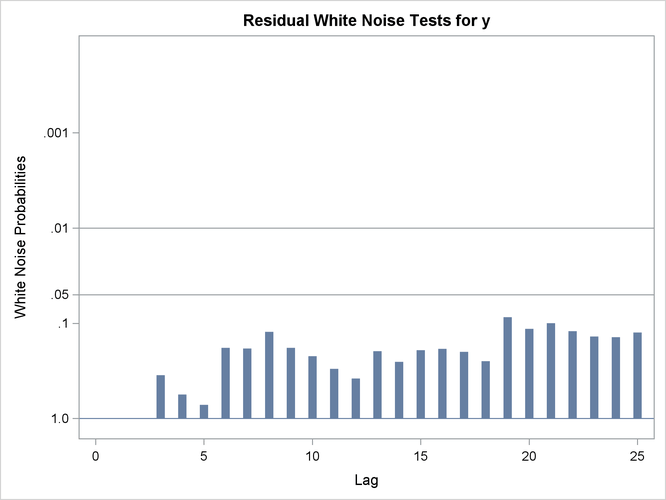Output 8.8.7: Q-Q Plot of Residuals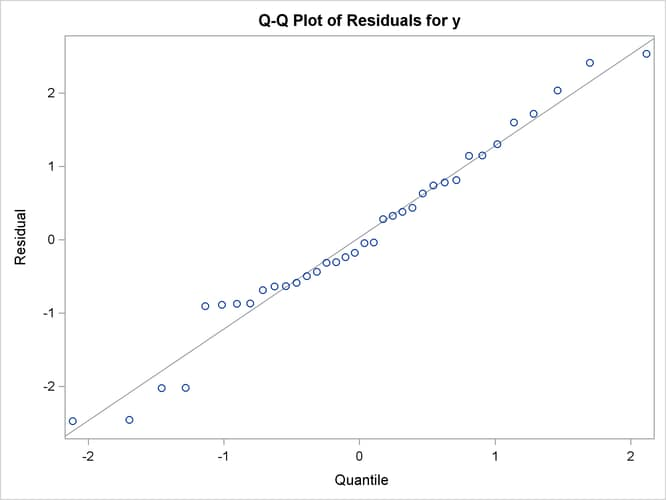Output 8.8.8: Histogram of Residuals